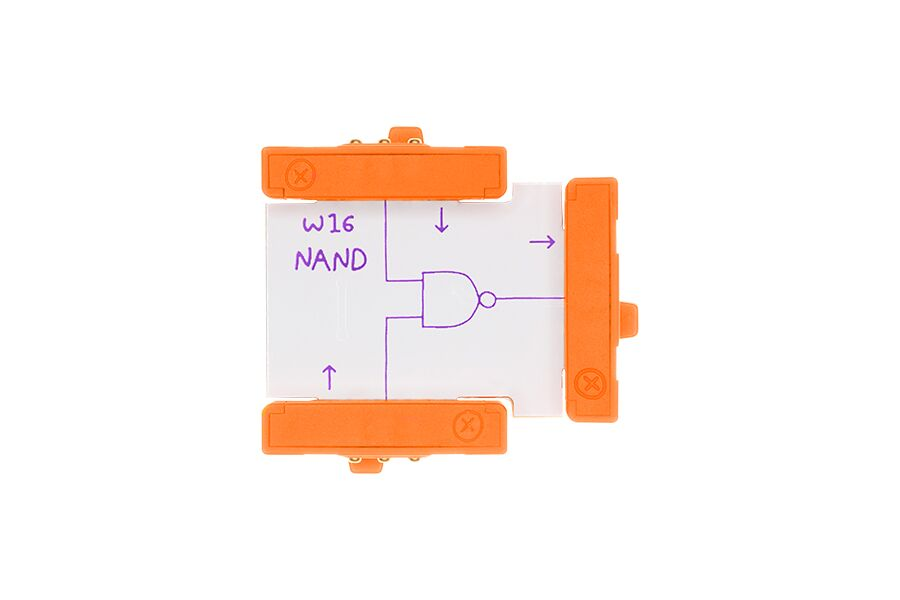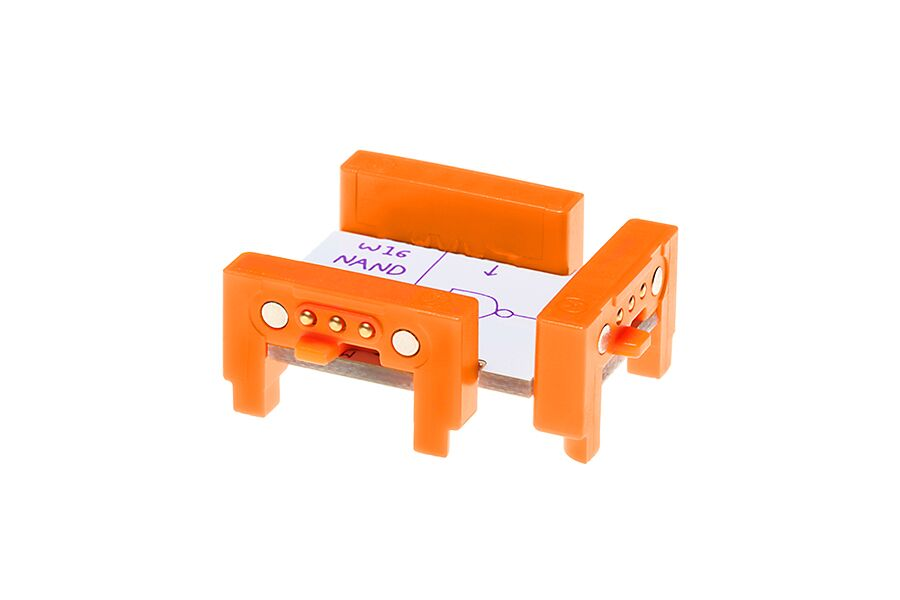The NAND Bit is a logic gate with 2 inputs. Think of it as "not and." The NAND Bit will always send an ON signal unless both input 1 and input 2 are receiving an ON signal. It’s the exact opposite of the AND Bit.

Sold out
• Meet the Bit
• Product Specs
• How it Works
• Community Inventions
• Kits with this Bit

The NAND Bit is a logic gate with two inputs and one output. NAND is short for NOT AND which is the combination of an AND gate where the output is inverted. The NAND only outputs an OFF signal when both of the inputs are ON, or true. If both inputs are OFF, the output signal will be ON. Building circuits with logic Bits is essentially the equivalent of physical prograqmming. Logic Bits create rules for your circuit to follow, giving you more ability to create interesting and complex interactions.

Users
Sizes
 Height 0.75" Width 5.0" Length 8.0"
Weight
 0.0072 kg/ 0.016 lbs
Model No.
 650-0086
UPC
810876022767

Anytime the NAND receives a signal lower than 2.5 volts at either of its inputs, the NAND sends an ON signal of 5 volts from its output. If the NAND receives a signal greater than 2.5 volts at either of its inputs, the NAND sends an ON signal of 5 volts from its output. If the NAND receives a signal greater than 2.5 volts at both of its inputs at the same time, the NAND sends an OFF signal of 0 volts from its output. Logic Bits like the NAND Bit only work with two states of inputs, ON and OFF. When an input is either ON or OFF, like a button, a toggle switch, or a sound trigger, we call it a digital input. Inputs that have variable voltage like pressure sensors, dimmers, and light sensors, are analog inputs. Because logic gates only deal with ON/OFF, high/low, or true/false, analog signals pass as digital. With analog inputs, an “ON” signal occurs at about 2.5 volts when passing through a logic gate. Also in logic circuits, you may want to hold the state of a momentary input (like a button). In order to hold this state, you can use a latch. You can also use a timeout to hold the state for a certain period of time.

# GET INSPIRED

## Find more than a thousand invention ideas and then share what you invent on littleBits Classroom. Here are just a few:

This should be inventions specific to this bit

# littleBits kits that include this bit:

#### MEET THE BIT

The NAND Bit is a logic gate with two inputs and one output. NAND is short for NOT AND which is the combination of an AND gate where the output is inverted. The NAND only outputs an OFF signal when both of the inputs are ON, or true. If both inputs are OFF, the output signal will be ON. Building circuits with logic Bits is essentially the equivalent of physical prograqmming. Logic Bits create rules for your circuit to follow, giving you more ability to create interesting and complex interactions.

#### PRODUCT SPECS

Users
Sizes
 Height 0.75" Width 5.0" Length 8.0"
Weight
 0.0072 kg/ 0.016 lbs
Model No.
 650-0086
UPC
810876022767

#### HOW IT WORKS

Anytime the NAND receives a signal lower than 2.5 volts at either of its inputs, the NAND sends an ON signal of 5 volts from its output. If the NAND receives a signal greater than 2.5 volts at either of its inputs, the NAND sends an ON signal of 5 volts from its output. If the NAND receives a signal greater than 2.5 volts at both of its inputs at the same time, the NAND sends an OFF signal of 0 volts from its output. Logic Bits like the NAND Bit only work with two states of inputs, ON and OFF. When an input is either ON or OFF, like a button, a toggle switch, or a sound trigger, we call it a digital input. Inputs that have variable voltage like pressure sensors, dimmers, and light sensors, are analog inputs. Because logic gates only deal with ON/OFF, high/low, or true/false, analog signals pass as digital. With analog inputs, an “ON” signal occurs at about 2.5 volts when passing through a logic gate. Also in logic circuits, you may want to hold the state of a momentary input (like a button). In order to hold this state, you can use a latch. You can also use a timeout to hold the state for a certain period of time.